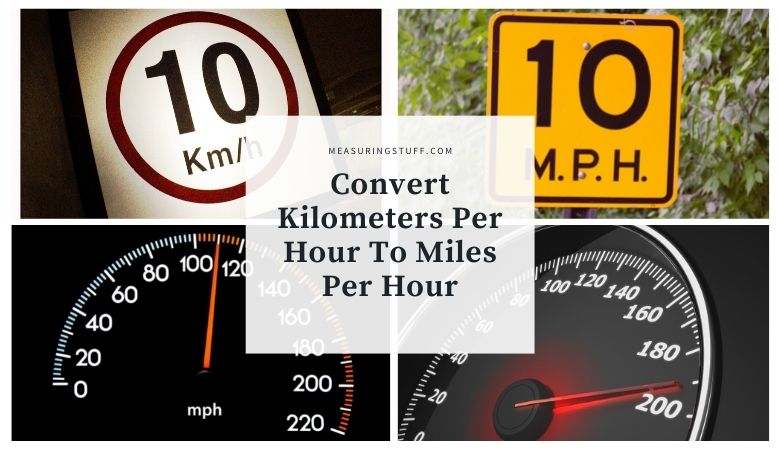# Convert Kilometers Per Hour To Miles Per Hour (KPH to MPH)

Converting kilometers per hour into miles per hour can be done easily by using the following formula.

There are 0.62137119223733 miles per hour in 1 kilometer per hour.

Simply multiply any kilometers per hour by 0.62137119223733 to convert into miles per hour.

For example, 1 KPH x 0.62137119223733 = 0.62137119223733 MPH.

Another example:

Convert 120 KPH into MPH.

120 x 0.62137119223733 = 74.564 MPH.

Some people will round off the multiple to 0.62 for easier math without a calculator handy.

Converting miles per hour into kilometers per hour can be done by multiplying the MPH by 1.609344.

For example, 1 MPH x 1.609344 = 1.609344 KPH.

Another example:

Convert 120 MPH into KPH.

120 x 1.609344 = 193.121 KPH.

You can also refer to the following table to convert KPH into MPH and vice versa.

### Kilometers per hour to miles per hour conversion calculator

Use the following calculator to easily convert KPH into MPH.

## How many KPH is 100 MPH?

100 miles per hour equals 160.92694 kilometers per hour.

Multiply 100 by 1.609344 to convert MPH into KPH.

100 x 1.609344 = 160.92694

## How many MPH is 100 KPH?

100 kilometers per hour equals 62.14000 miles per hour.

Multiply 100 by 0.62137119223733 to convert KPH into MPH.

100 x 0.62137119223733 = 62.14000

For our complete section of unit conversions including tables and calculators click the link here.